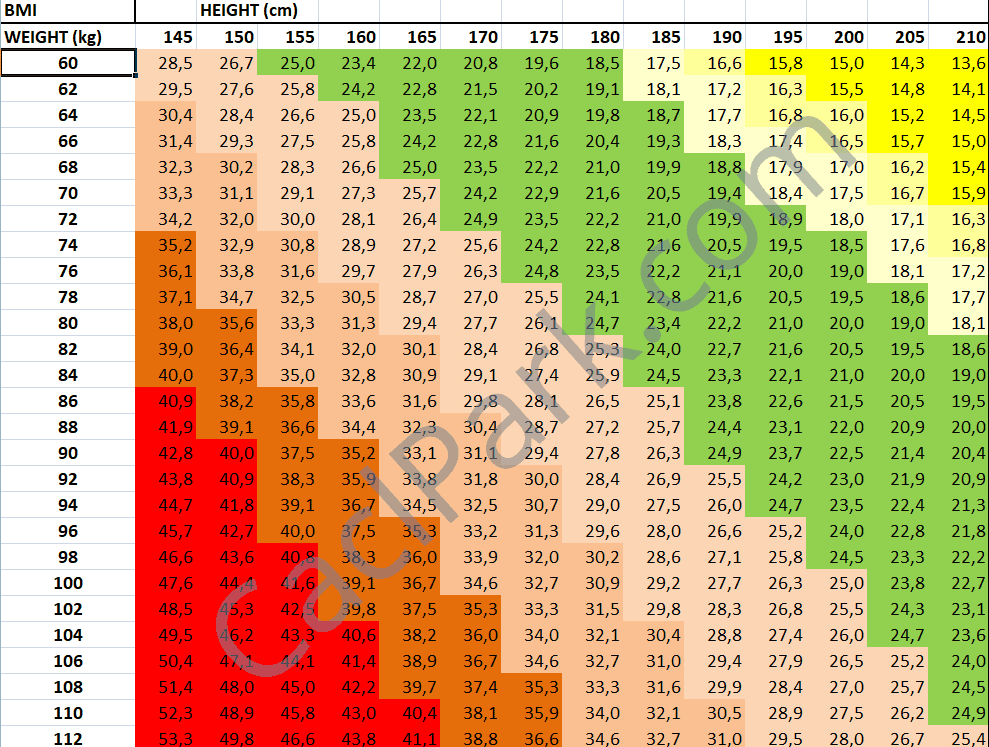# BMI Calculator

Calculator

Would you like to know whether your body is optimal, you are overweight or underweight based on your height and weight? Our body mass index (BMI) calculator helps you to find out.

The Body Mass Index (BMI) Calculator can be used to determine whether you are in a healthy weight range for your height.

## Example BMI-index calculations: METRIC UNITS

 BMI-index for 189 cm and 71 kg = 19.88 BMI-index for 182 cm and 72 kg = 21.74 BMI-index for 164 cm and 78 kg = 29 BMI-index for 151 cm and 61 kg = 26.75

## Example BMI-index calculations: US UNITS

 BMI-index for 5 ft 14 in and 143 lbs BMI-index for 5 ft 1 in and 188 lbs BMI-index for 5 ft 3 in and 197 lbs BMI-index for 5 ft 5 in and 186 lbs
Q&A

## 5 things worth knowing about your body mass index (BMI) and the BMI Calculator

1. BMI is the abbreviation for body mass index.

2. In the last couple of decades, BMI was frequently criticized, although this index has been used since the middle of the 19th century. Mathematicians of the Oxford University claim that the formula distorts reality and it is worth using a factor of 1.3 for the calculation. It corrects the fact that the standard BMI index evaluates taller people to be thicker while shorter ones to be thinners.

3. The classic body mass index does not show your body fat percentage.

4. The general formula of BMI index is not applicable to children. In general, the formula gives a plausible result over the age of 20, while it strongly distorts reality under the age of 18.

5. The formula used for the calculation of your body mass index is as follows

## How is BMI-index calculated?

Measurement UnitsFormula and Calculation
Kilograms and meters (or centimeters)Formula: weight (kg) / [height (m)]2

With the metric system, the formula for BMI is weight in kilograms divided by height in meters squared. Because height is commonly measured in centimeters, divide height in centimeters by 100 to obtain height in meters.

Example: Weight=68 kg, Height=165 cm (1.65 m)
Calculation: 68 ÷ (1.65)2=24.98

Pounds and inchesFormula: weight (lb) / [height (in)]2 x 703

Calculate BMI by dividing weight in pounds (lbs) by height in inches (in) squared and multiplying by a conversion factor of 703.

Example: Weight=150 lbs, Height=5’5″ (65″)
Calculation: [150 ÷ (65)2] x 703=24.96

## Body mass index table (metric units, kg and cm) presented by BMI Calculator## How is BMI interpreted for adults?

For adults 20 years old and older, BMI is interpreted using standard weight status categories. These categories are the same for men and women of all body types and ages.

 Body mass index (kg/m²) Weight category < 16 severly underweight 16 – 16,99 moderatly underweight 17 – 18,49 slightly underweight 18,5 – 24,99 normal weight 25 – 29,99 overweight 30 – 34,99 obese class I 35 – 39,99 obese class II ≥ 40 obese class III (severe)